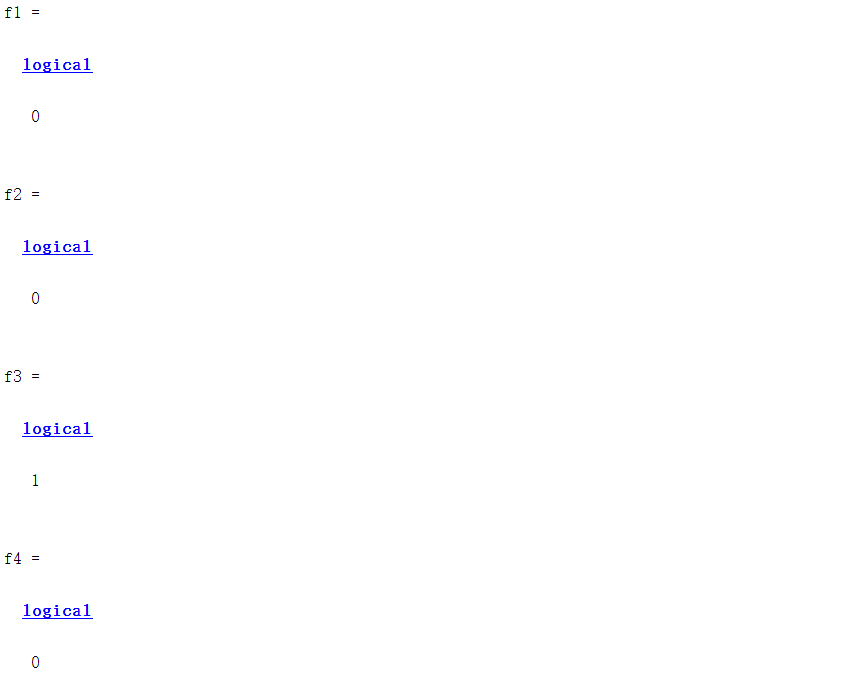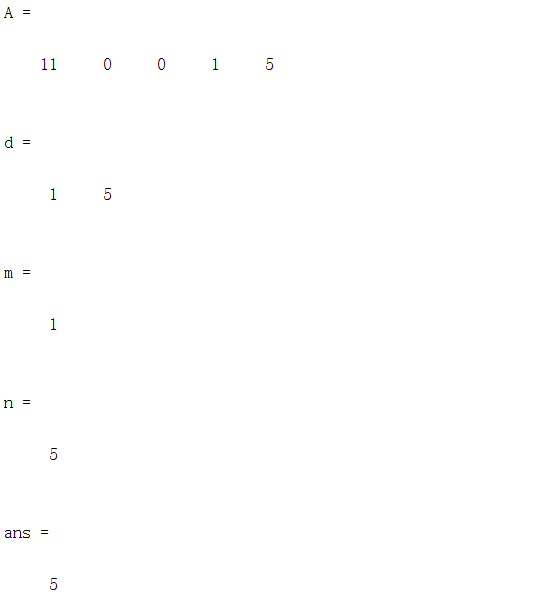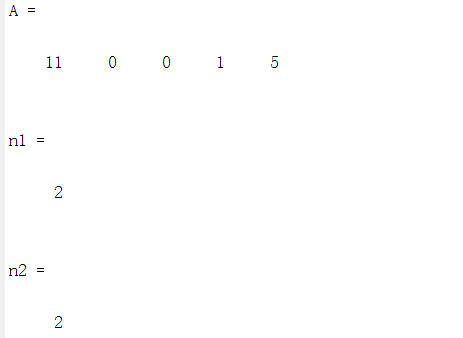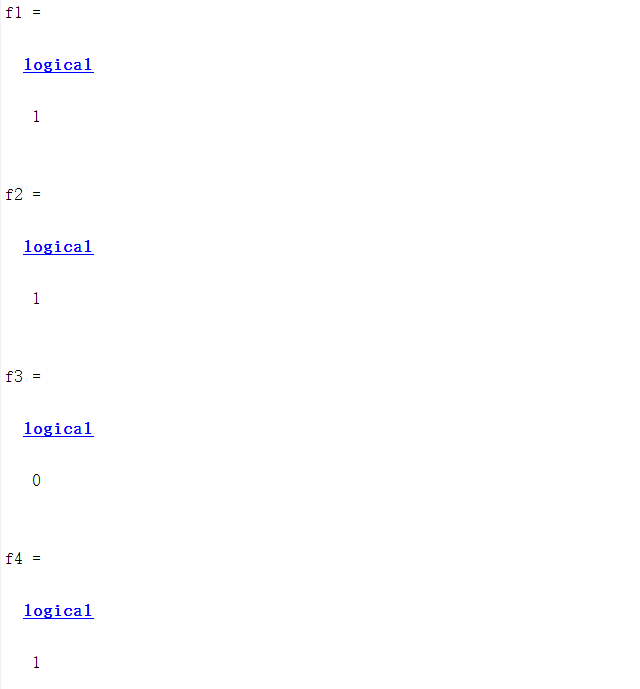# 数组信息的获取

1、函数isempty(A):检测数组是否为空数组，如果是空数组则返回值为“1”，否则返回值为“0”。
2、函数isscalar(A)检测数组是否为单个元素的标量。
3、函数isvector(A)检测数组是否为行向量或列向量。
4、函数isrow()检测数组是否为列向量。
5、函数iscolum()检测数组是否为行向量。
6、函数issparse()检测数组是否为稀疏矩阵。

clear all;
A=[11 0 0 1 5]
f1=isempty(A)
f2=isscalar(A)
f3=isvector(A)
f4=issparse(A)接下来我们来看看

clear all;
A=[11 0 0 1 5]
d=size(A)
[m,n]=size(A)
length(A)clear all;
A=[11 0 0 1 5]
n1=ndims(A)
a=5;
n2=ndims(a)clear all;
A=[10 9 6 1 5]
find(A>5)
find(A==5)
A(find(A>5))=A(find(A>5))+100
A(A==5)=200


clear all;
A=[11 0 0 1 5]
B=A>5
f1=isnumeric(A)
f2=isreal(A)
f3=isinteger(A)
f4=islogical(B)# 总结03-1009-14
03-22
10-07
08-02
04-25
08-22204
07-04133